# Frank Solutions for Class 10 Maths Chapter 13 Equation of a Straight Line

Frank Solutions for Class 10 Maths Chapter 13 Equation of a Straight Line are given here in a simple and comprehensive way. These solutions are extremely helpful for students to clear all their doubts easily and understand the basics of the chapter more effectively. Students of Class 10 are suggested to practise Frank Solutions for Class 10 Maths to strengthen the fundamentals and be able to solve questions that are usually asked in the examination.

Chapter 13 – Equation of a Straight Line is the common relation between the x- coordinates and y-coordinates of any point on the line.

## Download the PDF of Frank Solutions for Class 10 Maths Chapter 13 Equation of a Straight Line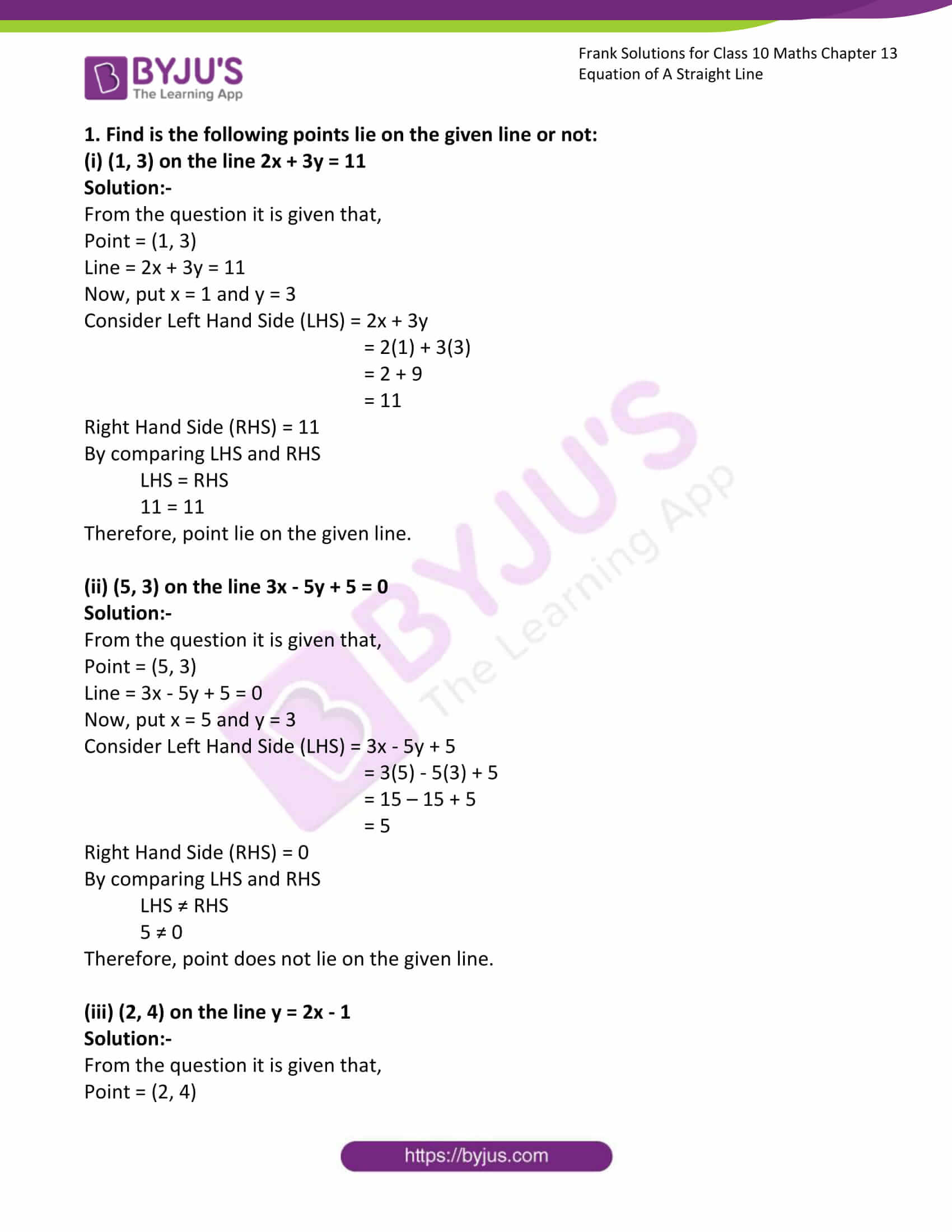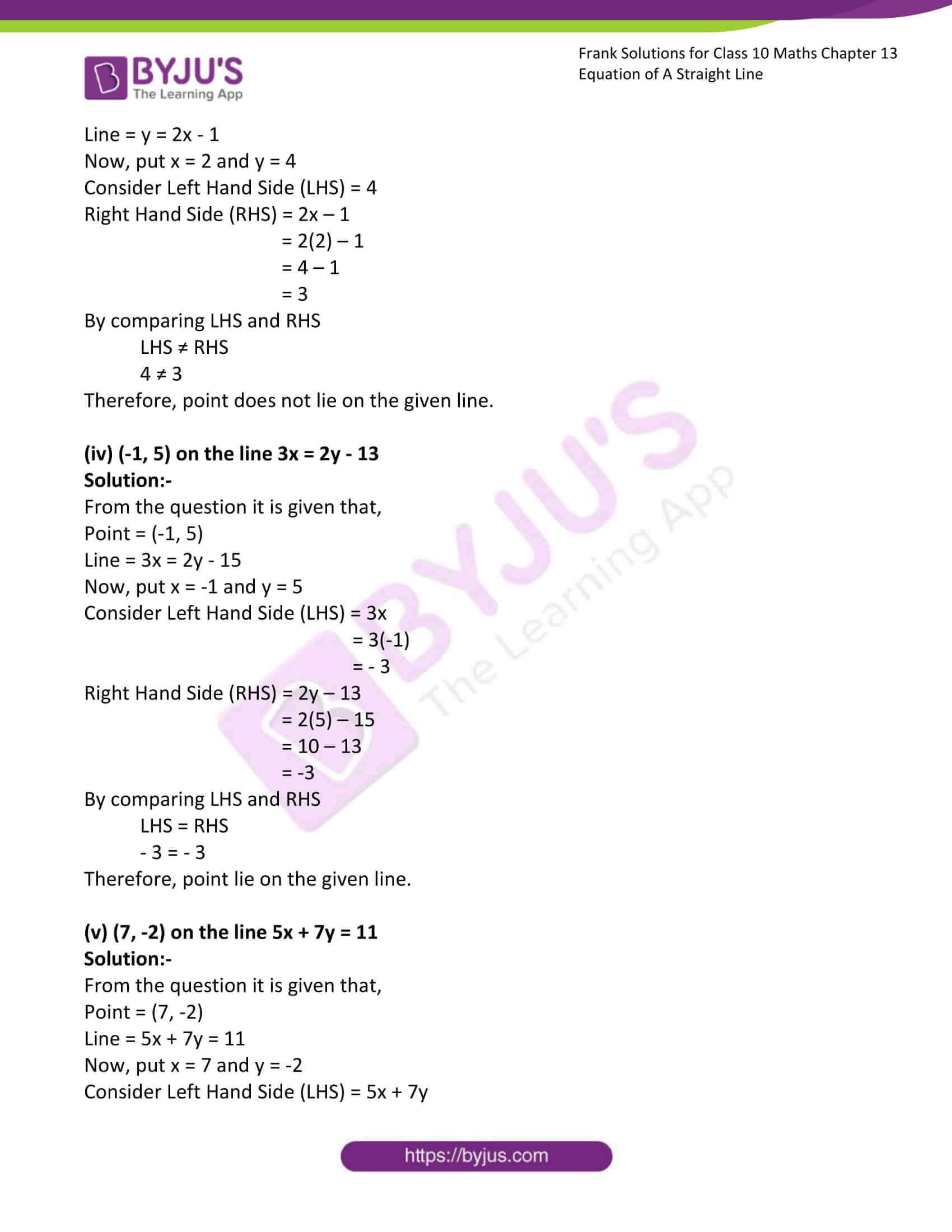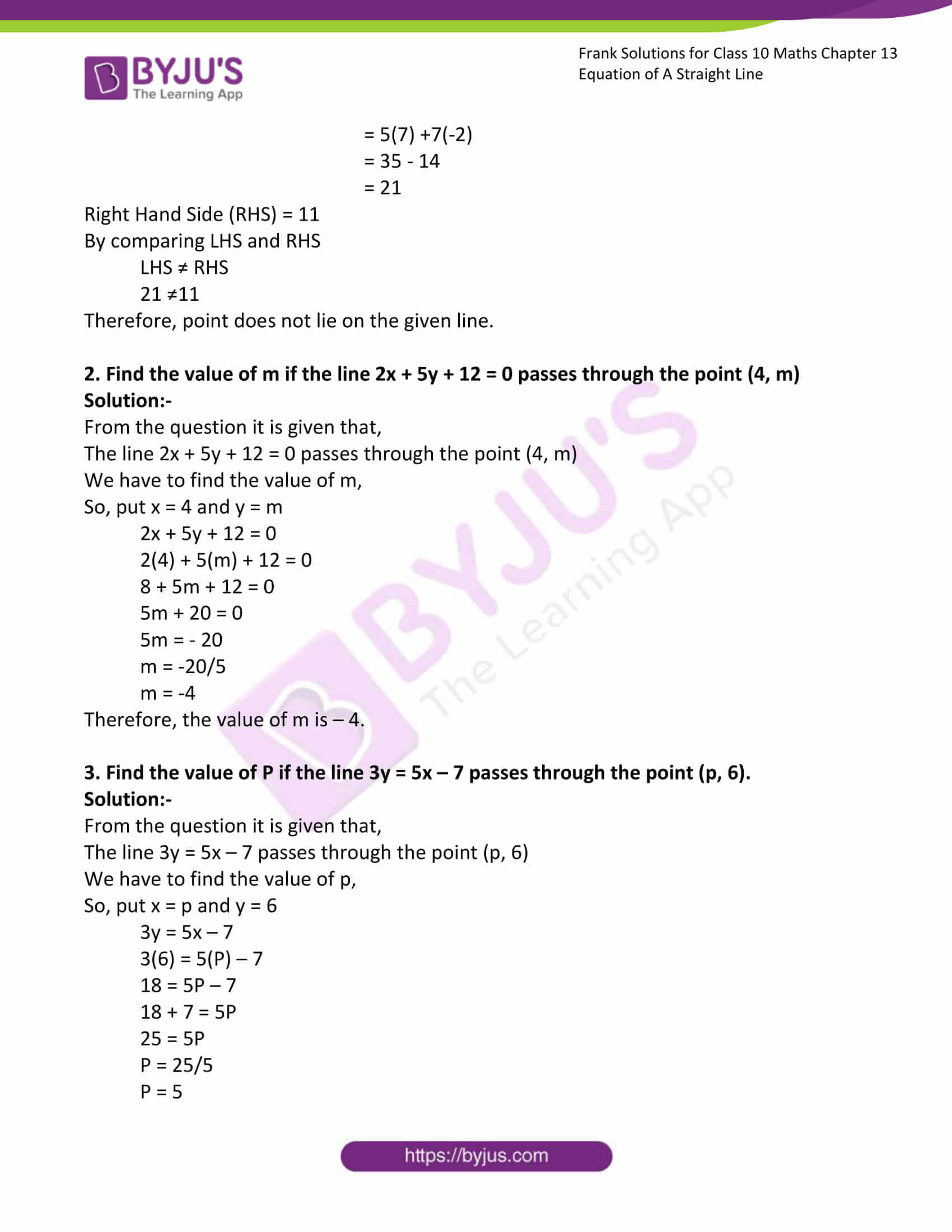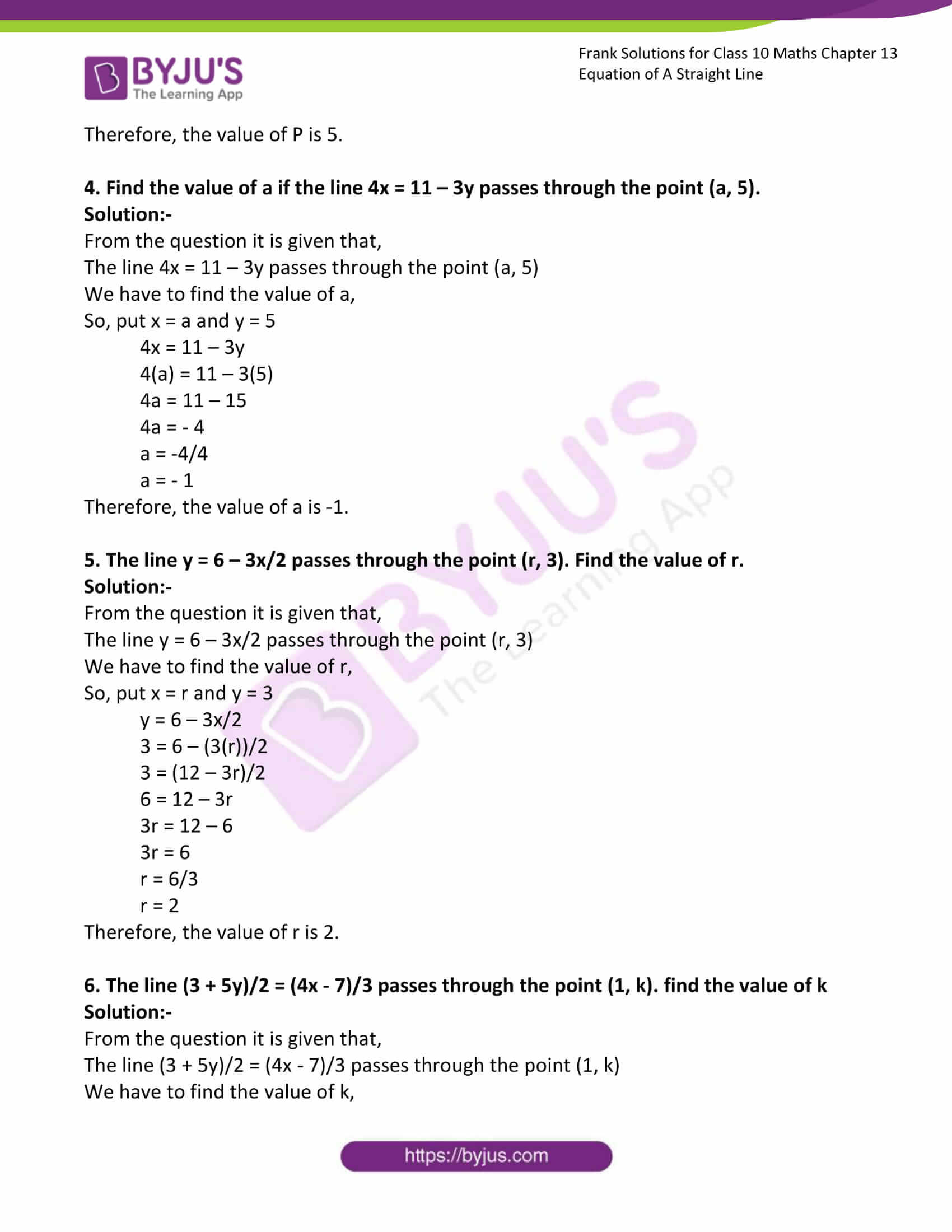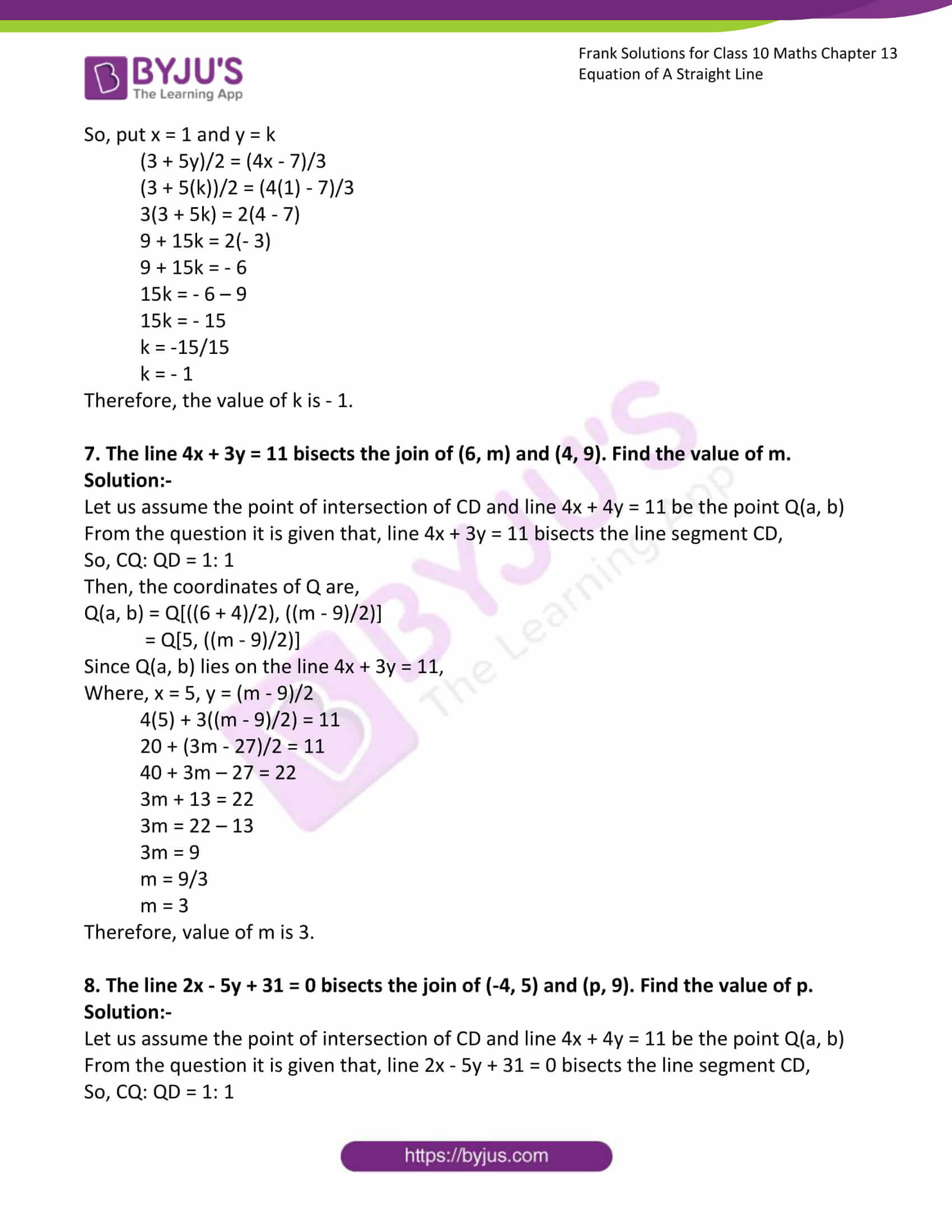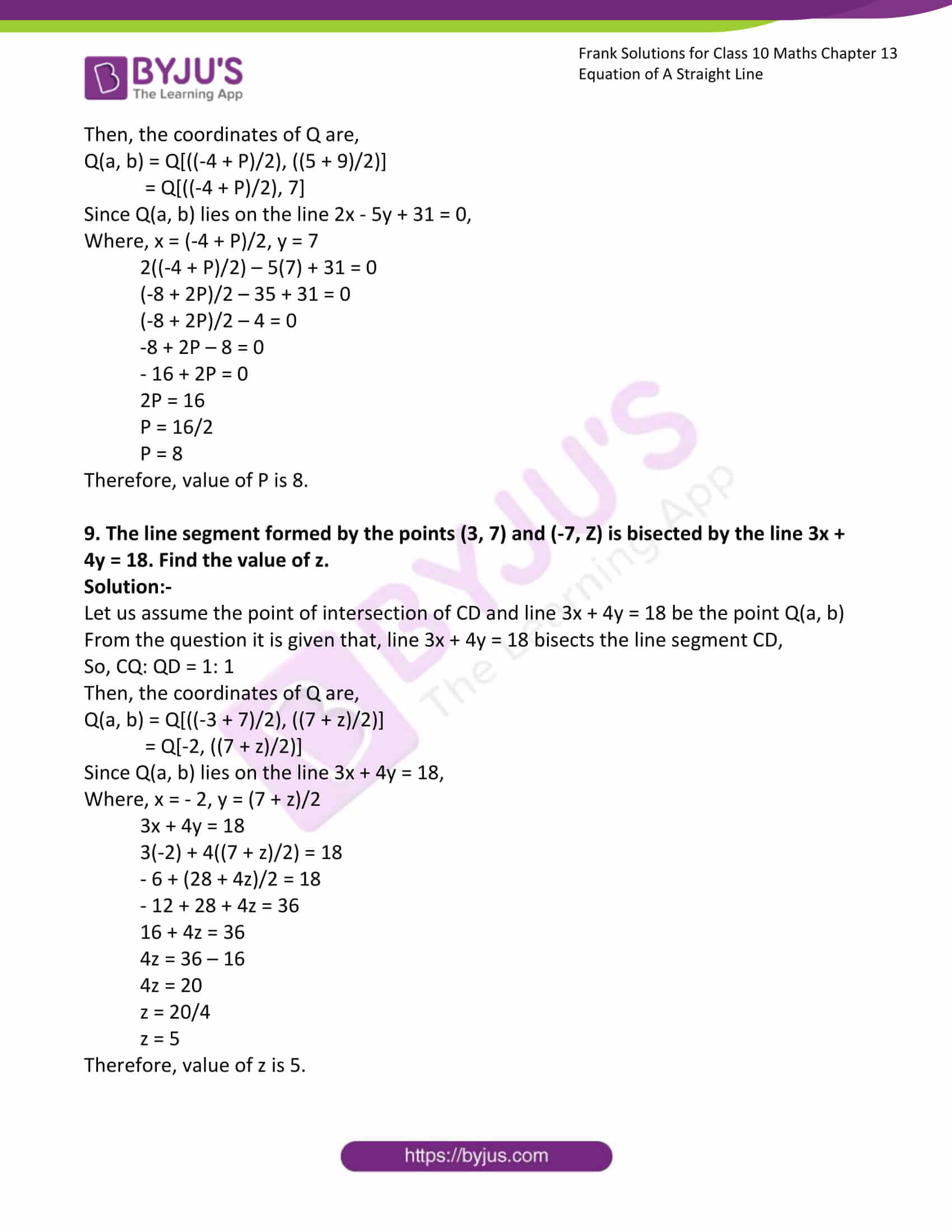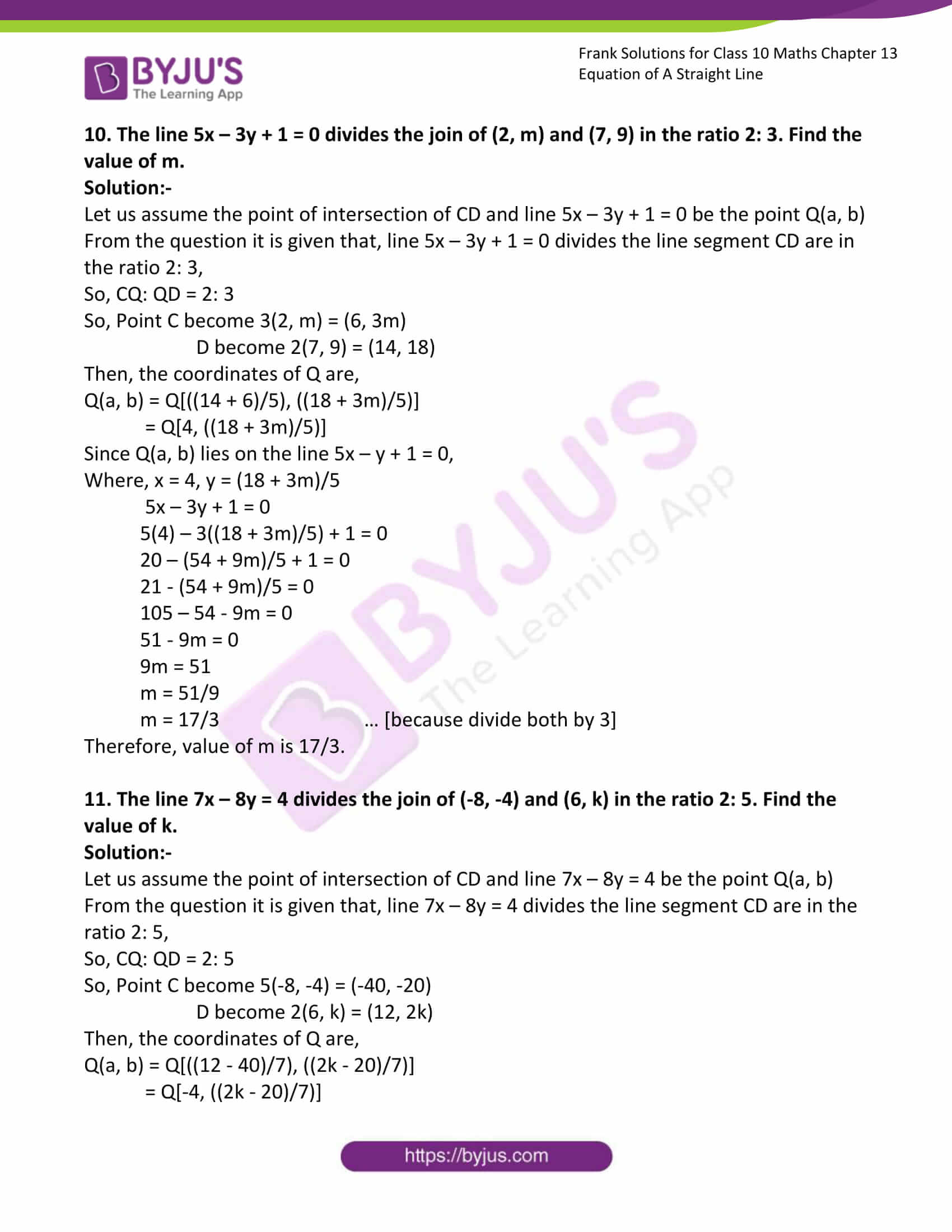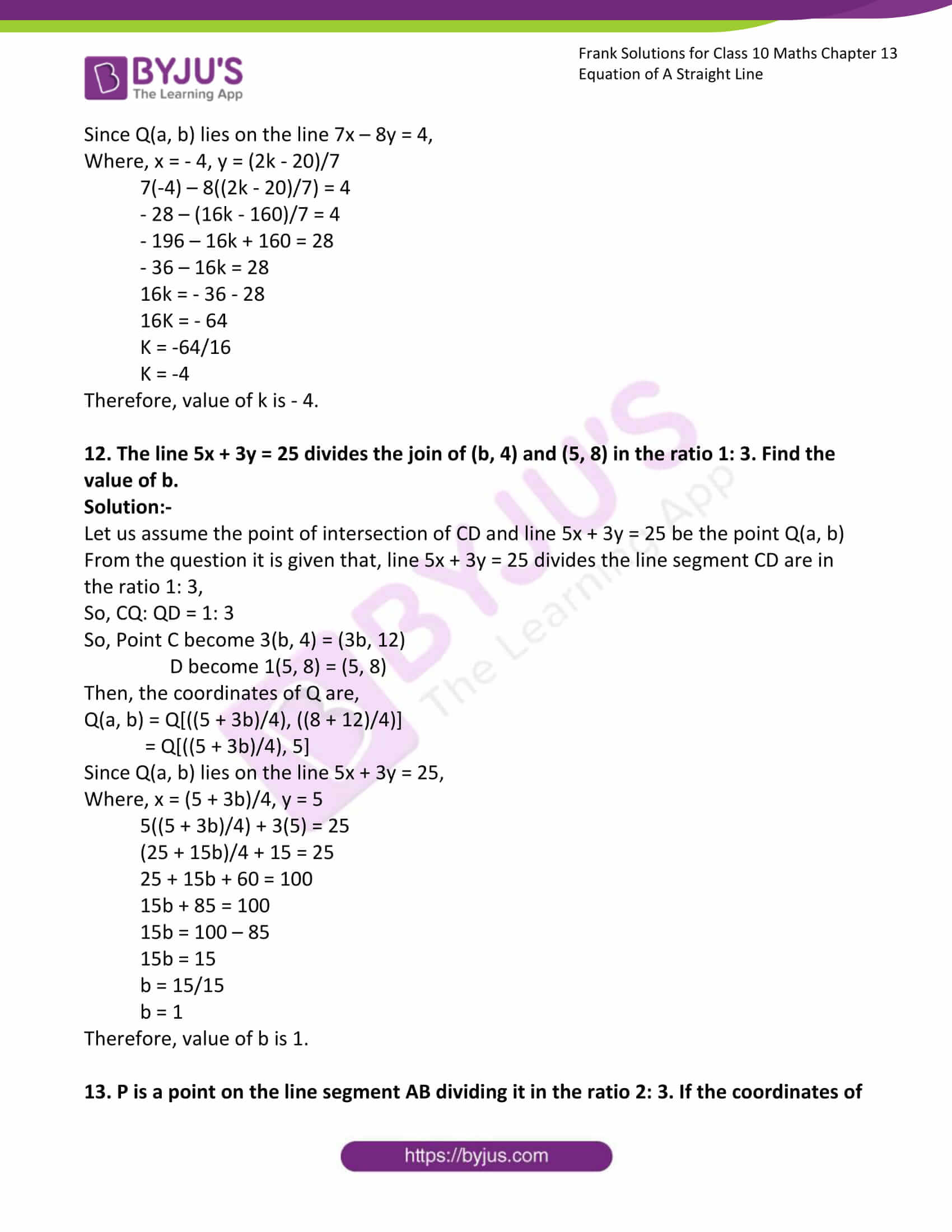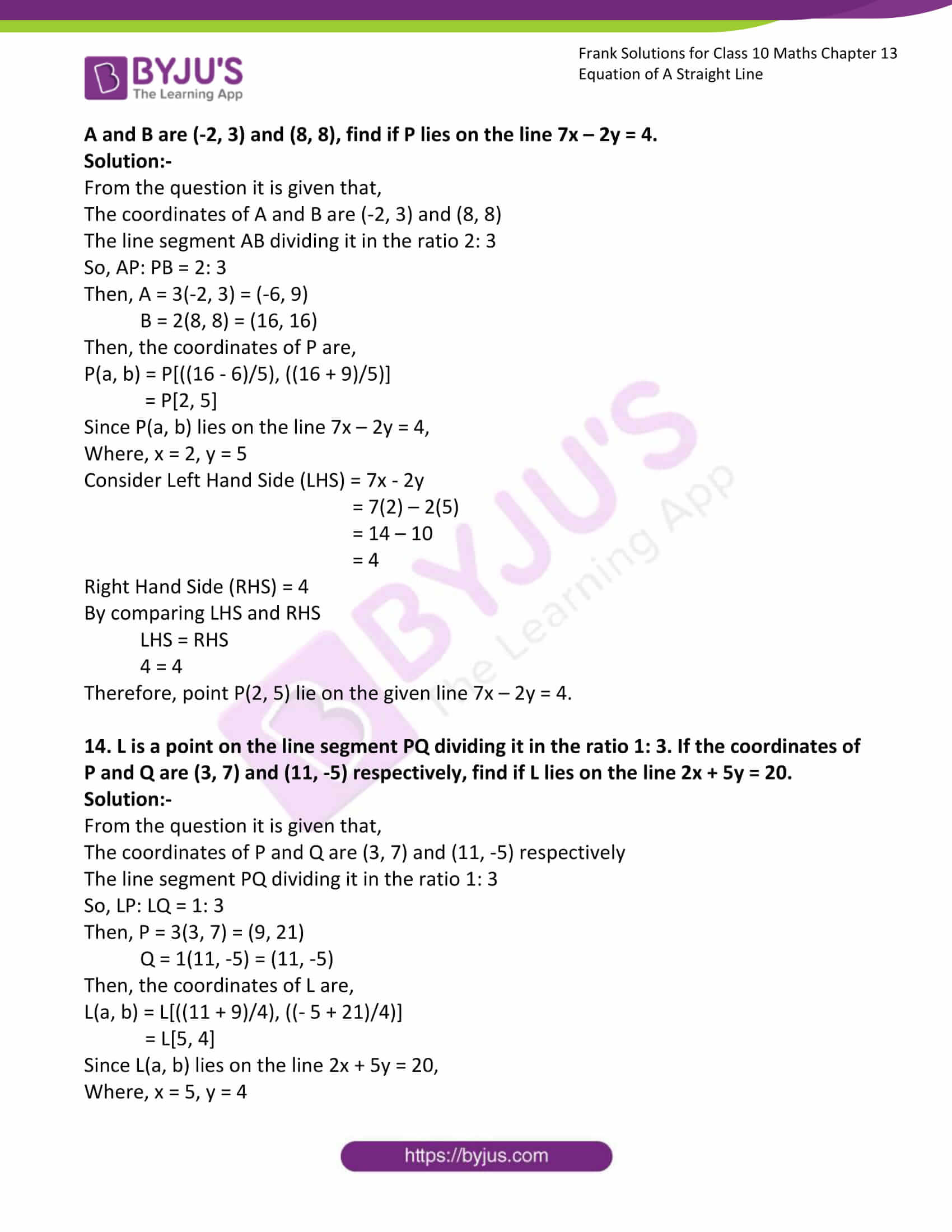### Access Answers to Frank Solutions for Class 10 Maths Chapter 13 Equation of a Straight Line

1. Find is the following points lie on the given line or not:

(i) (1, 3) on the line 2x + 3y = 11

Solution:-

From the question, it is given that,

Point = (1, 3)

Line = 2x + 3y = 11

Now, put x = 1 and y = 3

Consider Left Hand Side (LHS) = 2x + 3y

= 2(1) + 3(3)

= 2 + 9

= 11

Right Hand Side (RHS) = 11

By comparing LHS and RHS

LHS = RHS

11 = 11

Therefore, point lie on the given line.

(ii) (5, 3) on the line 3x – 5y + 5 = 0

Solution:-

From the question, it is given that,

Point = (5, 3)

Line = 3x – 5y + 5 = 0

Now, put x = 5 and y = 3

Consider Left Hand Side (LHS) = 3x – 5y + 5

= 3(5) – 5(3) + 5

= 15 – 15 + 5

= 5

Right Hand Side (RHS) = 0

By comparing LHS and RHS

LHS ≠ RHS

5 ≠ 0

Therefore, the point does not lie on the given line.

(iii) (2, 4) on the line y = 2x – 1

Solution:-

From the question, it is given that,

Point = (2, 4)

Line = y = 2x – 1

Now, put x = 2 and y = 4

Consider Left Hand Side (LHS) = 4

Right Hand Side (RHS) = 2x – 1

= 2(2) – 1

= 4 – 1

= 3

By comparing LHS and RHS,

LHS ≠ RHS

4 ≠ 3

Therefore, point does not lie on the given line.

(iv) (-1, 5) on the line 3x = 2y – 13

Solution:-

From the question, it is given that,

Point = (-1, 5)

Line = 3x = 2y – 15

Now, put x = -1 and y = 5

Consider Left Hand Side (LHS) = 3x

= 3(-1)

= – 3

Right Hand Side (RHS) = 2y – 13

= 2(5) – 15

= 10 – 13

= -3

By comparing LHS and RHS,

LHS = RHS

– 3 = – 3

Therefore, point lie on the given line.

(v) (7, -2) on the line 5x + 7y = 11

Solution:-

From the question, it is given that,

Point = (7, -2)

Line = 5x + 7y = 11

Now, put x = 7 and y = -2

Consider Left Hand Side (LHS) = 5x + 7y

= 5(7) +7(-2)

= 35 – 14

= 21

Right Hand Side (RHS) = 11

By comparing LHS and RHS

LHS ≠ RHS

21 ≠11

Therefore, the point does not lie on the given line.

2. Find the value of m if the line 2x + 5y + 12 = 0 passes through the point (4, m)

Solution:-

From the question, it is given that,

The line 2x + 5y + 12 = 0 passes through the point (4, m)

We have to find the value of m,

So, put x = 4 and y = m

2x + 5y + 12 = 0

2(4) + 5(m) + 12 = 0

8 + 5m + 12 = 0

5m + 20 = 0

5m = – 20

m = -20/5

m = -4

Therefore, the value of m is – 4.

3. Find the value of P if the line 3y = 5x – 7 passes through the point (p, 6).

Solution:-

From the question, it is given that,

The line 3y = 5x – 7 passes through the point (p, 6)

We have to find the value of p,

So, put x = p and y = 6

3y = 5x – 7

3(6) = 5(P) – 7

18 = 5P – 7

18 + 7 = 5P

25 = 5P

P = 25/5

P = 5

Therefore, the value of P is 5.

4. Find the value of a if the line 4x = 11 – 3y passes through the point (a, 5).

Solution:-

From the question, it is given that,

The line 4x = 11 – 3y passes through the point (a, 5)

We have to find the value of a,

So, put x = a and y = 5

4x = 11 – 3y

4(a) = 11 – 3(5)

4a = 11 – 15

4a = – 4

a = -4/4

a = – 1

Therefore, the value of a is -1.

5. The line y = 6 – 3x/2 passes through the point (r, 3). Find the value of r.

Solution:-

From the question, it is given that,

The line y = 6 – 3x/2 passes through the point (r, 3)

We have to find the value of r,

So, put x = r and y = 3

y = 6 – 3x/2

3 = 6 – (3(r))/2

3 = (12 – 3r)/2

6 = 12 – 3r

3r = 12 – 6

3r = 6

r = 6/3

r = 2

Therefore, the value of r is 2.

6. The line (3 + 5y)/2 = (4x – 7)/3 passes through the point (1, k). find the value of k

Solution:-

From the question, it is given that,

The line (3 + 5y)/2 = (4x – 7)/3 passes through the point (1, k)

We have to find the value of k,

So, put x = 1 and y = k

(3 + 5y)/2 = (4x – 7)/3

(3 + 5(k))/2 = (4(1) – 7)/3

3(3 + 5k) = 2(4 – 7)

9 + 15k = 2(- 3)

9 + 15k = – 6

15k = – 6 – 9

15k = – 15

k = -15/15

k = – 1

Therefore, the value of k is – 1.

7. The line 4x + 3y = 11 bisects the join of (6, m) and (4, 9). Find the value of m.

Solution:-

Let us assume the point of intersection of CD and line 4x + 4y = 11 be the point Q(a, b)

From the question it is given that, line 4x + 3y = 11 bisects the line segment CD,

So, CQ: QD = 1: 1

Then, the coordinates of Q are,

Q(a, b) = Q[((6 + 4)/2), ((m – 9)/2)]

= Q[5, ((m – 9)/2)]

Since Q(a, b) lies on the line 4x + 3y = 11,

Where, x = 5, y = (m – 9)/2

4(5) + 3((m – 9)/2) = 11

20 + (3m – 27)/2 = 11

40 + 3m – 27 = 22

3m + 13 = 22

3m = 22 – 13

3m = 9

m = 9/3

m = 3

Therefore, value of m is 3.

8. The line 2x – 5y + 31 = 0 bisects the join of (-4, 5) and (p, 9). Find the value of p.

Solution:-

Let us assume the point of intersection of CD and line 4x + 4y = 11 be the point Q(a, b)

From the question it is given that, line 2x – 5y + 31 = 0 bisects the line segment CD,

So, CQ: QD = 1: 1

Then, the coordinates of Q are,

Q(a, b) = Q[((-4 + P)/2), ((5 + 9)/2)]

= Q[((-4 + P)/2), 7]

Since Q(a, b) lies on the line 2x – 5y + 31 = 0,

Where, x = (-4 + P)/2, y = 7

2((-4 + P)/2) – 5(7) + 31 = 0

(-8 + 2P)/2 – 35 + 31 = 0

(-8 + 2P)/2 – 4 = 0

-8 + 2P – 8 = 0

– 16 + 2P = 0

2P = 16

P = 16/2

P = 8

Therefore, the value of P is 8.

9. The line segment formed by the points (3, 7) and (-7, Z) is bisected by the line 3x + 4y = 18. Find the value of z.

Solution:-

Let us assume the point of intersection of CD and line 3x + 4y = 18 be the point Q(a, b)

From the question it is given that, line 3x + 4y = 18 bisects the line segment CD,

So, CQ: QD = 1: 1

Then, the coordinates of Q are,

Q(a, b) = Q[((-3 + 7)/2), ((7 + z)/2)]

= Q[-2, ((7 + z)/2)]

Since Q(a, b) lies on the line 3x + 4y = 18,

Where, x = – 2, y = (7 + z)/2

3x + 4y = 18

3(-2) + 4((7 + z)/2) = 18

– 6 + (28 + 4z)/2 = 18

– 12 + 28 + 4z = 36

16 + 4z = 36

4z = 36 – 16

4z = 20

z = 20/4

z = 5

Therefore, the value of z is 5.

10. The line 5x – 3y + 1 = 0 divides the join of (2, m) and (7, 9) in the ratio 2: 3. Find the value of m.

Solution:-

Let us assume the point of intersection of CD and line 5x – 3y + 1 = 0 be the point Q(a, b)

From the question, it is given that line 5x – 3y + 1 = 0 divides the line segment CD are in the ratio 2: 3,

So, CQ: QD = 2: 3

So, Point C become 3(2, m) = (6, 3m)

D become 2(7, 9) = (14, 18)

Then, the coordinates of Q are,

Q(a, b) = Q[((14 + 6)/5), ((18 + 3m)/5)]

= Q[4, ((18 + 3m)/5)]

Since Q(a, b) lies on the line 5x – y + 1 = 0,

Where, x = 4, y = (18 + 3m)/5

5x – 3y + 1 = 0

5(4) – 3((18 + 3m)/5) + 1 = 0

20 – (54 + 9m)/5 + 1 = 0

21 – (54 + 9m)/5 = 0

105 – 54 – 9m = 0

51 – 9m = 0

9m = 51

m = 51/9

m = 17/3 … [because divide both by 3]

Therefore, the value of m is 17/3.

11. The line 7x – 8y = 4 divides the join of (-8, -4) and (6, k) in the ratio 2: 5. Find the value of k.

Solution:-

Let us assume the point of intersection of CD and line 7x – 8y = 4 be the point Q(a, b)

From the question, it is given that line 7x – 8y = 4 divides the line segment CD are in the ratio 2: 5,

So, CQ: QD = 2: 5

So, Point C become 5(-8, -4) = (-40, -20)

D become 2(6, k) = (12, 2k)

Then, the coordinates of Q are,

Q(a, b) = Q[((12 – 40)/7), ((2k – 20)/7)]

= Q[-4, ((2k – 20)/7)]

Since Q(a, b) lies on the line 7x – 8y = 4,

Where, x = – 4, y = (2k – 20)/7

7(-4) – 8((2k – 20)/7) = 4

– 28 – (16k – 160)/7 = 4

– 196 – 16k + 160 = 28

– 36 – 16k = 28

16k = – 36 – 28

16K = – 64

K = -64/16

K = -4

Therefore, the value of k is – 4.

12. The line 5x + 3y = 25 divides the join of (b, 4) and (5, 8) in the ratio 1: 3. Find the value of b.

Solution:-

Let us assume the point of intersection of CD and line 5x + 3y = 25 be the point Q(a, b)

From the question, it is given that line 5x + 3y = 25 divides the line segment CD are in the ratio 1: 3,

So, CQ: QD = 1: 3

So, Point C become 3(b, 4) = (3b, 12)

D become 1(5, 8) = (5, 8)

Then, the coordinates of Q are,

Q(a, b) = Q[((5 + 3b)/4), ((8 + 12)/4)]

= Q[((5 + 3b)/4), 5]

Since Q(a, b) lies on the line 5x + 3y = 25,

Where, x = (5 + 3b)/4, y = 5

5((5 + 3b)/4) + 3(5) = 25

(25 + 15b)/4 + 15 = 25

25 + 15b + 60 = 100

15b + 85 = 100

15b = 100 – 85

15b = 15

b = 15/15

b = 1

Therefore, the value of b is 1.

13. P is a point on the line segment AB dividing it in the ratio 2: 3. If the coordinates of A and B are (-2, 3) and (8, 8), find if P lies on the line 7x – 2y = 4.

Solution:-

From the question, it is given that,

The coordinates of A and B are (-2, 3) and (8, 8)

The line segment AB dividing it in the ratio 2: 3

So, AP: PB = 2: 3

Then, A = 3(-2, 3) = (-6, 9)

B = 2(8, 8) = (16, 16)

Then, the coordinates of P are,

P(a, b) = P[((16 – 6)/5), ((16 + 9)/5)]

= P[2, 5]

Since P(a, b) lies on the line 7x – 2y = 4,

Where, x = 2, y = 5

Consider Left Hand Side (LHS) = 7x – 2y

= 7(2) – 2(5)

= 14 – 10

= 4

Right Hand Side (RHS) = 4

By comparing LHS and RHS

LHS = RHS

4 = 4

Therefore, point P(2, 5) lie on the given line 7x – 2y = 4.

14. L is a point on the line segment PQ dividing it in the ratio 1: 3. If the coordinates of P and Q are (3, 7) and (11, -5), respectively, find if L lies on the line 2x + 5y = 20.

Solution:-

From the question, it is given that,

The coordinates of P and Q are (3, 7) and (11, -5), respectively

The line segment PQ dividing it in the ratio 1: 3

So, LP: LQ = 1: 3

Then, P = 3(3, 7) = (9, 21)

Q = 1(11, -5) = (11, -5)

Then, the coordinates of L are,

L(a, b) = L[((11 + 9)/4), ((- 5 + 21)/4)]

= L[5, 4]

Since L(a, b) lies on the line 2x + 5y = 20,

Where, x = 5, y = 4

Consider Left Hand Side (LHS) = 2x + 5y

= 2(5) + 5(4)

= 10 + 20

= 30

Right Hand Side (RHS) = 20

By comparing LHS and RHS

LHS ≠ RHS

30 ≠ 20

Therefore, point L(a, b) does not lie on the given line 2x + 5y = 20.

15. The line segment formed by two points A(2, 3) and B(5, 6) is divided by a point in the ratio 1: 2. Find, whether the point of intersection lies on the line 3x – 4y + 5 = 0.

Solution:-

From the question, it is given that,

The coordinates of A(2, 3) and B(5, 6).

The line segment AB dividing it in the ratio 1: 2

So, AL: LB = 1: 3

Then, A = 2(2, 3) = (4, 6)

B = 1(5, 6) = (5, 6)

Then, the coordinates of L are,

L(a, b) = L[((5 + 4)/3), ((6 + 6)/3)]

= L[3, 4]

Since L(a, b) lies on the line 3x – 4y + 5 = 0,

Where, x = 3, y = 4

Consider Left Hand Side (LHS) = 3x – 4y + 5

= 3(3) – 4(4) + 5

= 9 – 16 + 5

= – 2

Right Hand Side (RHS) = 0

By comparing LHS and RHS

LHS ≠ RHS

-2 ≠ 0

Therefore, point L(a, b) does not lie on the given line 3x – 4y + 5 = 0.